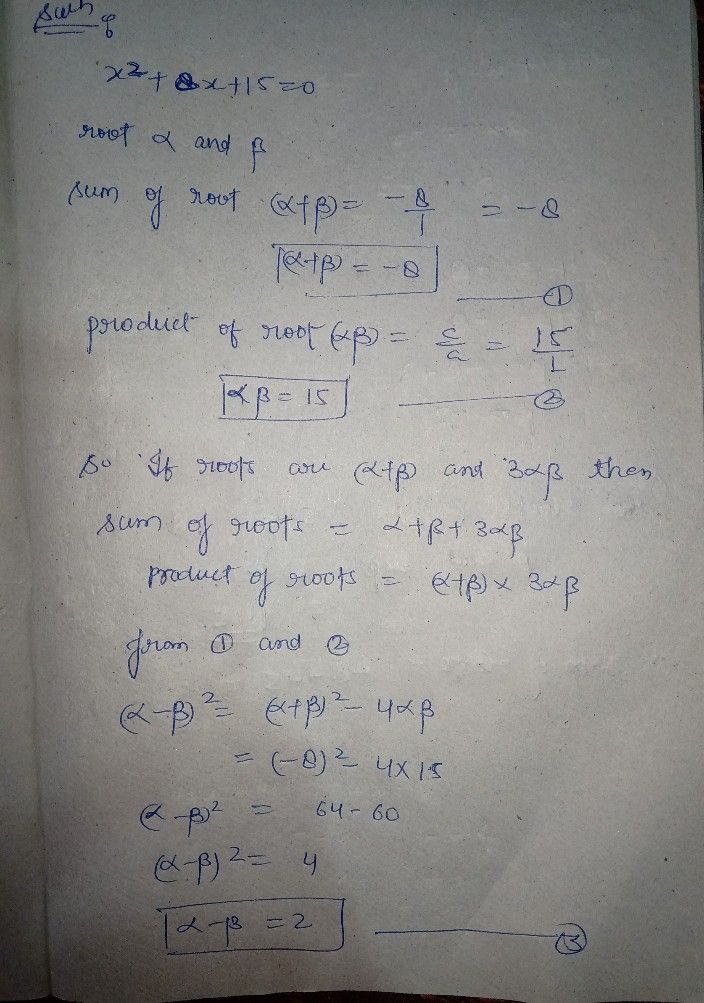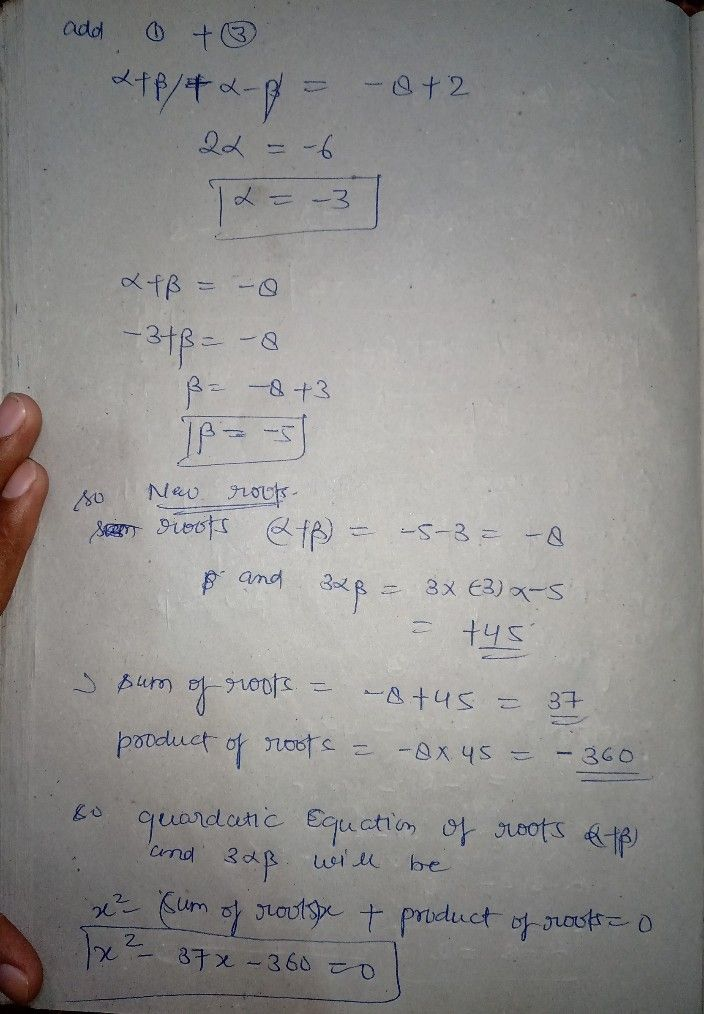Symbol
Problem$8.$ If a andB $β$ are the roots of the equation $x^{2}+8x+15=0$ then form the equation whose roots $arQ$ $\left(α+β\right)$ and $3αβ$ $○$ O a). $\right).$ $x^{2}-35x-300=0$ $○$ O b). $\right).x^{2}-37x-360=0$ $○$ O c). $\right).x^{2}-30x+360=0$
10th-13th grade
Algebra
SolutionQanda teacher - Rahulrajptdear
I upload remaing answer
just 1 minStudent
ok MadamQanda teacher - Rahulrajptoption B is correct ...
if u face any doubt in this question plz askStudent
Thank you Madam
sure....Qanda teacher - Rahulrajpt
aAnd if u satishfy plz evaluate with good ratings